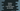# Four different methods to check if all items are similar in python list## Check if all items are similar in python list :

In this python tutorial, we will learn how to check if all items are similar or not in a python list.For example, for the list [1,1,1,1,1], all items are same but for [1,2,1,1,1], all items are not same.We have different ways to solve this problem in python. In this post, I will show you four different methods to solve it. Let’s take a look at them :

The source code is available here.

### Method 1: Using a loop :

This is the most used process by anyone. Run one loop through the list and compare the elements one by one like below :

In this example, we have stored the first element of the list in a variable and compare it with all other elements in the list. We have one separate function is_all_items_unique to do the checking. If any element is not the same as the first element, it returns False. Else returns True. Based on the return value, print out the output to the user.

The above program will print out the following output :

### Method 2 : Using count() :

The list.count(value) method takes one parameter value and calculates the count of it in the list. So, if all elements of a list are unique, list.count(list) will be equal to the length of the list. We can easily implement this concept by comparing the value of count() for the first element of the list with the length of the list len(list).

It will print out the same output as the above example.

### Method 3 : Using set() :

We know that a set contains only unique elements. We can create a set by passing a list as a parameter to set() constructor. It will create one new set by removing all duplicate elements from the list. So, if all the elements of our list are unique, the size of the set will be 1, isn’t it? Let’s check it :

Here, mainly we are checking the length of the set is 1 or not. If 1, means all elements are the same.

### Method-4 : Using all() :

all method takes an iterable as input and returns True if all values are True for the iterable. So, we can optimize our first solution using this method. That means we will pass one iterable to check if all elements are the same as the first element or not. Based on that, we will print out the result as the above examples :

### Conclusion :

We have learnt four different methods to find out if a list contains the same element or not in python. I hope you have found something useful in this program. If you have any queries, drop a comment below and don’t forget to subscribe to our newsletter. Happy coding :)